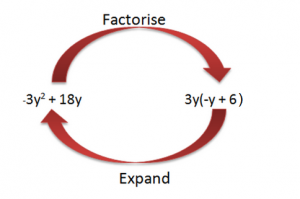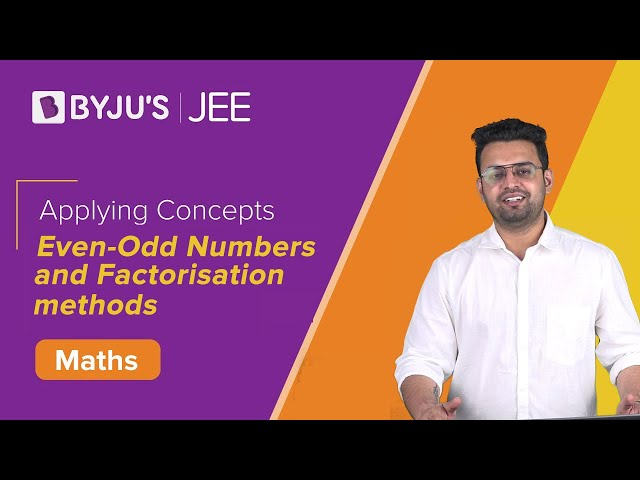Checkout JEE MAINS 2022 Question Paper Analysis : Checkout JEE MAINS 2022 Question Paper Analysis :

# Factorisation Of Algebraic Expression: Algebra

A number or quantity that when multiplied with another number produces a given number or expression. For example, the factors of 12 are 1, 2, 3, 4, 6 and 12.

12 = 1 × 12

12 = 2 × 6

12 = 3 × 4

Any number can be expressed in the form of its factors as shown above.

In terms of its prime factors, 12 can be expressed as:

12 = 2 × 3 × 2

Similarly, an algebraic expression can also be expressed in the form of its factors. An algebraic expression consists of variables, constants and operators. An algebraic expression consists of terms separated by an addition operation. Consider the following algebraic expression:

$$\begin{array}{l}3xyz – 16x^{2} -yz\end{array}$$

This expression consists of 3 terms

$$\begin{array}{l}3xyz\end{array}$$
$$\begin{array}{l}- 16x^{2}\end{array}$$
and
$$\begin{array}{l}-yz\end{array}$$
. Each term of this algebraic expression can be expressed in the form of its factors as:

$$\begin{array}{l}3xyz = 3.x.y.z\end{array}$$
,
$$\begin{array}{l}- 16x^{2} = -1.2.2.2.2.x.x\end{array}$$
and
$$\begin{array}{l}-yz = -1 . y . z\end{array}$$
.

Algebraic Expressions can be factorized using many methods. The most common methods used for factorization of algebraic expressions are:

• Factorization using common factors
• Factorization by regrouping terms
• Factorization using identities

Let us discuss these methods one by one in detail:

## Factorization using common factors

To factorize an algebraic expression, the highest common factors of the terms of the given algebraic expression are determined and then we group the terms accordingly. In simple terms, the reverse process of expansion of an algebraic expression is its factorization.

To understand this more clearly let us take an example.

Example-

$$\begin{array}{l}-3y^{2} + 18y\end{array}$$

Solution- The algebraic expression can be re-written as

$$\begin{array}{l}-3y^{2} + 18y = -3.y.y + 3.6.y\end{array}$$

$$\begin{array}{l}\Rightarrow -3y^{2} + 18y = -3.y(y – 6)\end{array}$$Consider the algebraic expression 3y(- y + 6), if we expand this we will get -3y2 + 18y.

## Factorization by regrouping terms

In some algebraic expressions, not every term may have a common factor. For instance, consider the algebraic expression 12a + n -na – 12. The terms of this expression do not have a particular factor in common but the first and last term has a common factor of ‘12’ similarly second and third term has n as a common factor. So the terms can be regrouped as:

⇒12a + n – na – 12= 12a – 12 + n – an

⇒12a – 12 – an + n = 12(a -1) –n(a -1)

After regrouping it can be seen that (a-1) is a common factor in each term,

⇒12a + n -na – 12=(a-1) (12 – n)

Thus, by regrouping terms we can factorize algebraic expressions.

## Factorizing Expressions using standard identities

An equality relation which holds true for all the values of variables in mathematics is known as an identity. Consider the following identities:

$$\begin{array}{l}(a+b)^{2} = a^{2}+b^{2}+2ab\end{array}$$

$$\begin{array}{l}(a-b)^{2} = a^{2}+b^{2}-2ab\end{array}$$

$$\begin{array}{l}a^{2}-b^{2} = (a+b) (a-b)\end{array}$$

On substituting any value of a and b, both sides of the given equations remain the same. Therefore, these equations are called identities.

Example: Factorize

$$\begin{array}{l}9x^{2} + 4m^{2} + 12mx\end{array}$$
.

Solution: Observe the given expression carefully. This expression has three terms and all the terms are positive. Moreover, the first and the second term are perfect squares. The expression fits the form

$$\begin{array}{l}(a+b)^{2} = a^{2}+b^{2}+2ab\end{array}$$
where a = 3x, b = 2m.

$$\begin{array}{l}9x^{2} + 4m^{2} + 12mx = (3x)^{2} + (2m)^{2} +2. 3x . 2m\end{array}$$

Therefore,

$$\begin{array}{l}9x^{2} + 4m^{2} + 12mx = (3x+2m)^{2}\end{array}$$

Thus, the required factorization of 9x2+ 4m2 + 12mx is (3x + 2m)2 using standard identities.

## Video Lesson

### FactorisationQuiz on Factorisation Of Algebraic Expression

1. Anekwe Miranda

Like this

site. It’s educative

2. Piyush Pandey

Thanks for this answer . It is really helpful for me !

3. PS Pratham